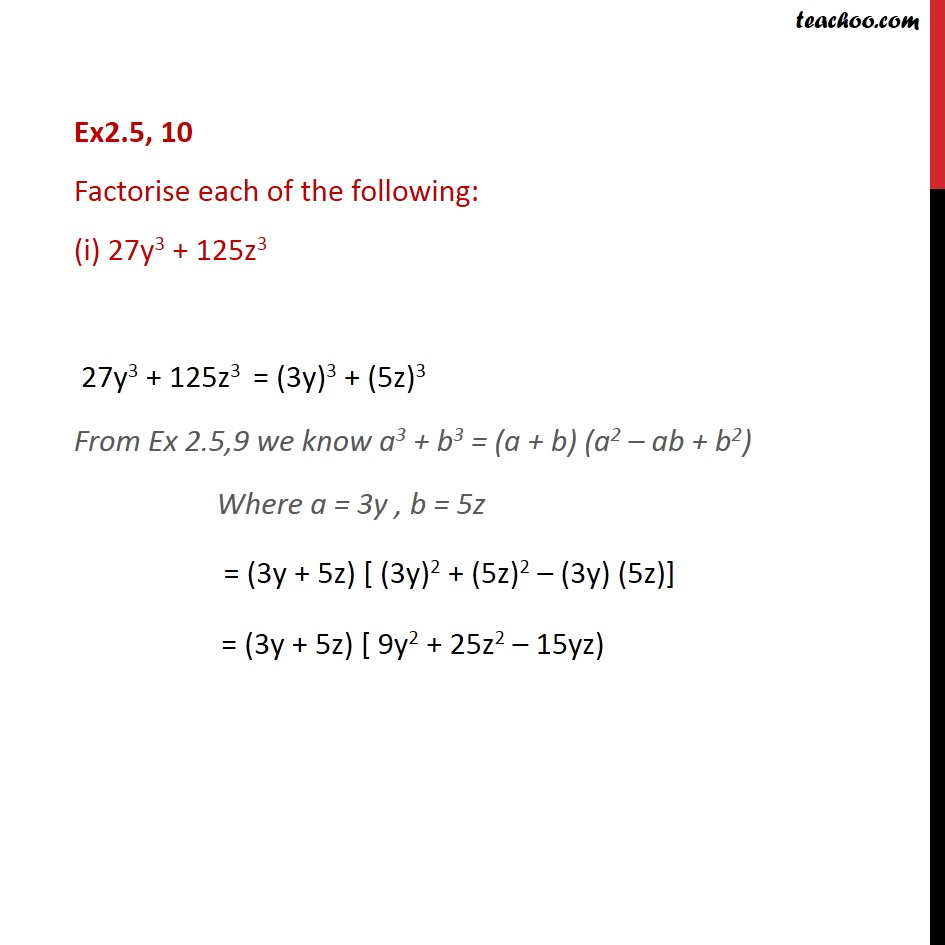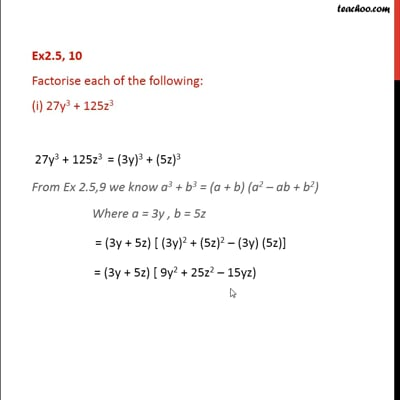Identity VIII

Chapter 2 Class 9 Polynomials (Term 2)
Concept wiseThis video is only available for Teachoo black users

### Transcript

Ex2.5, 10 Factorise each of the following: (i) 27y3 + 125z3 27y3 + 125z3 = (3y)3 + (5z)3 From Ex 2.5,9 we know a3 + b3 = (a + b) (a2 – ab + b2) Where a = 3y , b = 5z = (3y + 5z) [ (3y)2 + (5z)2 – (3y) (5z)] = (3y + 5z) [ 9y2 + 25z2 – 15yz)